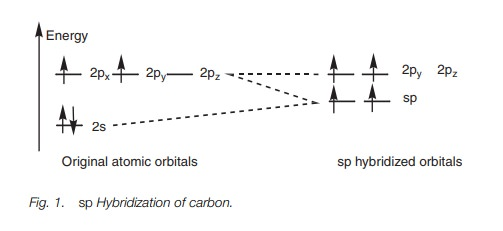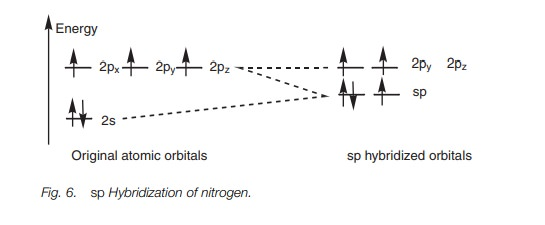Home | | Organic Chemistry | sp Hybridization

# sp HybridizationIn sp hybridization, the 2s orbital and one of the three 2p orbitals are ‘mixed’ to form two hybridized sp orbitals of equal energy. Two 2p orbitals are left over and have slightly higher energy than the unhybridized orbitals.

SP HYBRIDIZATION

## Definition

In sp hybridization, the 2s orbital and one of the three 2p orbitals are ‘mixed’ to form two hybridized sp orbitals of equal energy. Two 2p orbitals are left over and have slightly higher energy than the unhybridized orbitals.

## Electronic configuration

For carbon, each sp hybridized orbital contains a single unpaired electron. There are also two half-filled 2p orbitals. Therefore, four bonds are possible.

## Geometry

Each sp orbital is shaped like a deformed dumbbell with one lobe much larger than the other. The remaining 2p orbitals are symmetrical dumbbells. If we define the 2p orbitals as being aligned along the y and the z axes, the two sp hybridized orbitals point in opposite directions along the x axis.

## Alkynes

Each sp hybridized carbon of an alkyne can form two σ bonds using sp hybridized orbitals. The remaining 2p orbitals can overlap ‘side-on’ to form two πbonds. Alkynes are linear molecules and are reactive due to the π bonds.

## Nitrile groups

The nitrogen and carbon atoms of a nitrile group (C==N) are both sp hybridized. The carbon has two sp hybridized orbitals and can form two σ bonds, one of which is to nitrogen. The nitrogen has one sp orbital which is used in the σ bond with carbon. Both the carbon and the nitrogen have two 2p orbitals which can be used to form two π bonds.

## Definition

In sp hybridization, the 2s orbital is mixed with one of the 2p orbitals (e.g. 2px) to give two sp hybrid orbitals of equal energy. This leaves two 2p orbitals unaffected (2py and 2pz) with slightly higher energy than the hybridized orbitals (Fig. 1).## Electronic configuration

For carbon, the first two electrons fit into each sp orbital according to Hund’s rule such that each orbital has a single unpaired electron. This leaves two electrons which can be paired up in the half-filled sp orbitals or placed in the vacant 2py and 2pz orbitals. The energy difference between the orbitals is small and so it is easier for the electrons to fit into the higher energy orbitals than to pair up. This leads to two half-filled sp orbitals and two half-filled 2p orbitals (Fig. 1), and so four bonds are possible.

## Geometry

The  2p  orbitals  are  dumbbell  in  shape  while  the  sp  hybridized  orbitals  are deformed dumbbells with one lobe much larger than the other. The 2pand 2porbitals are at right angles to each other (Fig. 2a). The sp hybridized orbitals occupy the space left over and are in the x axis pointing in opposite directions (only the major lobe of the sp orbitals are shown in black; Fig. 2b).A molecule using the two sp orbitals for bonding will be linear in shape. There are two common functional groups where such bonding takes place – alkynes and nitriles.

## Alkynes

Let us consider the bonding in ethyne (Fig. 3) where each carbon is sp hybridized. The C–H bonds are strong σ bonds where each hydrogen atom uses its half-filled 1s orbital to bond with a half-filled sp orbital on carbon.The remaining sp orbital on each carbon is used to form a strong σ carbon–carbon bond. The full σ bonding diagram for ethyne is linear (Fig. 4a) and can be simplified as shown (Fig. 4b).Further bonding is possible since each carbon has half-filled p orbitals. Thus, the 2py and 2pz orbitals of each carbon atom can overlap side-on to form two π bonds (Fig. 5). The π bond formed by the overlap of the 2py orbitals is represented in dark gray. The π bond resulting from the overlap of the 2pz orbitals is represented in light gray. Alkynes are linear molecules and are reactive due to the relatively weak bonds.## Nitrile groups

Exactly the same theory can be used to explain the bonding within a nitrile group (C == N) where both the carbon and the nitrogen are sphybridized. The energy level diagram in Fig. 6 shows how the valence electrons of nitrogen are arranged after sp hybridization. A lone pair of electrons occupies one of the sp orbitals, but the other sp orbital can be used for a strong σ bond. The 2py and 2pz orbitals can be used for two π bonds. Figure 7 represents the σ bonds of HCN as lines and how the remaining 2p orbitals are used to form two π bonds.Study Material, Lecturing Notes, Assignment, Reference, Wiki description explanation, brief detail
Organic Chemistry: Structure and bonding : sp Hybridization |